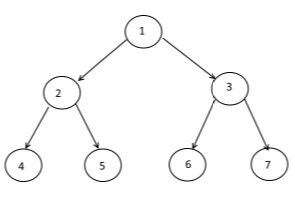# Pre-order Inorder and Post Order traversal of a Binary Tree

suggest change

Consider the Binary Tree:Pre-order traversal(root) is traversing the node then left sub-tree of the node and then the right sub-tree of the node.

So the pre-order traversal of above tree will be:

1 2 4 5 3 6 7

In-order traversal(root) is traversing the left sub-tree of the node then the node and then right sub-tree of the node.

So the in-order traversal of above tree will be:

4 2 5 1 6 3 7

Post-order traversal(root) is traversing the left sub-tree of the node then the right sub-tree and then the node.

So the post-order traversal of above tree will be:

4 5 2 6 7 3 1